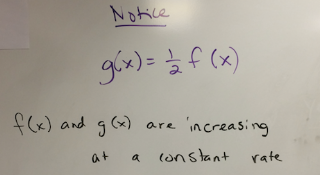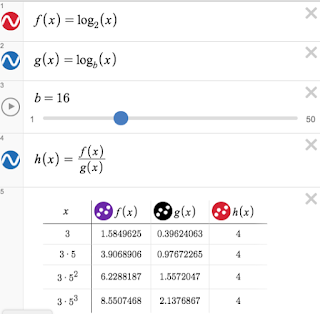## Tuesday, March 20, 2018

### Teach 180: Becoming Mathematicians (Day 122)

On Day 117, I posted that I wanted to take a class period to have my students look at the following problem.  You can read more about the blog post here.

My students have never formally worked with geometric or arithmetic sequences before and I thought that I might use this problem to introduce them to those ideas.  But as my ideas for the lesson took form, I decided that I wanted them to play with the mathematics and that too much formality might squash that.  Here are the 7 steps that were part of that discovery.

Here are two main items that my students noticed.  For some reason I was so focused on the relationship between the log of a geometric sequence and the resulting arithmetic sequence that I didn't notice the very first thing that my students noticed! (Note: The bases of 2 and 4 were chosen to make it easier for students to make observations.)Here is what my students wondered:

1) Is the ratio of f(x):g(x) always 2?

2) What if a and r weren't even, positive integers?

3) What happens if 0 < r < 1?

Here are screenshots from Desmos to show some of the student's investigations.This screenshot shows one instance where a and r (initial term and constant ratio) are positive odd integers.  Students noted that the ratio of log2 x and log4 x is still 2.  Then, the question becomes "why?" We proved this was true by rewriting log2 x and log4 x with change of base formula.  We had log2 x = (log x)/(log 2) and log4 x = (log x)/(log 4).  After writing the quotient of f(x)/g(x) and simplifying, we had (log 4)/(log 2).  Since 4 can be thought of as 2 squared, log 4 can be rewritten as log 22 or 2(log 2). So, 2(log 2)/(log 2) = 2.Next, we investigated how to use a slider to look at many different values for the base.  At one point, my students were even able to predict what values of b would produce a ratio that is an integer.  They recognized that since f(x) was log base 2, that b would need to be a power of 2. We also looked at how the table of values changed if r was a value between 0 and 1.  This led to an arithmetic sequence that was decreasing by a constant difference, instead of increasing by a constant difference.
Although we did not get around to creating a more formal proof, I was pleased that my students felt comfortable with making observations, not knowing for sure if there was a right answer and checking their conjectures both informally (with desmos) and more formally with properties of logarithms.  We finished class with me telling my students that they really were "thinking like mathematicians" that making observations, forming conjectures and deciding if they are true or not was what it really means to "do math".  One of my students noted that Desmos made it much easier to easily test our ideas.